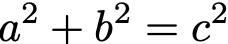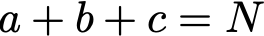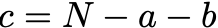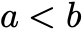# 2 quick lessons from a C# coding exercise

This simple problem reminded me not to overthink — unless you have to.

### 2 quick lessons from a C# coding exercise

This simple problem reminded me not to overthink — unless you have to.I still like doing coding exercises, even after years of programming. I recently came across this neat exercise — a simplified Pythagorean triplet— that reminded me not to overthink problems until you really have to.

Fair warning —this is not a hard problem. But I want to remember these takeaways:

(1) You don’t always need to do a lot of work to figure out a good complexity algorithm for a problem. Sometimes we want to grab our favorite data structures right away, but sometimes also reworking the problem can cut down the complexity.

(2) A good guess is often good enough — e.g. working out better ranges for loops is sometimes not even worth it, and even a simple guess will suffice until we really have to solve it.

### The problem

The Pythagorean triple is three positive integers `a,b,c` that satisfy:A common coding exercise is to find all `a,b,c` that are each less than `N` that satisfy this equation (emphasis on ).

A simplified version of this problem is to consider another condition:This addition greatly simplifies the problem, and a very efficient algorithm can be easily found.

Problem statement: for a given `N` find all `a,b,c` that satisfy both equations.

### Think about getting lower complexity

Obviously, do not write three for loops for each `a,b,c` and brute force check the conditions.

We have three variables `a,b,c` and 2 equations. This means that there is only one independent variable, and only one for loop needed. Since the algorithm just has a single loop searching for possible values of `a`, the time complexity of the algorithm is O(N).

Notice that we didn’t need to do any work for this — just from the problem setup we know there is only one loop.

Here is the skeleton in C#:

I put a simple bound here on the for loop of `N/3` .

We know this is a loose bound, because if all numbers were equal `a=b=c` then the second equation gives the bound on the lowest value `a` as `N/3` . We will revisit this at the end and get a better bound and see how bad our simple guess is.

### Finding b,c

Let’s take the second constraint and rearrange it for `c` :and then plug it into the first one to solve for `b` :Of course, in the code, we have to check that this results in an integer value — most combinations of `N,a` do not. Here is the implementation in C# of checking if there is a valid `b` parameter given `N` and `a` :

After that we can get `c = N — b — a` . Here is the resulting algorithm in C#:

### Getting a bound on our only for loop

Let’s get a bound on our for loop. We already have the bound `a < N/3` from before, but can we do better?

We can use the conditiontogether with the previous equation for `b` to arrive at:This was solved by applying the quadratic formula — from it we get two solutions for the bound, but we can just take the smaller one.

#### Why don’t we have to check that b < c?

Since we used the condition `a^2 + b^2 = c^2` explicitly in finding `b` and `c`, then the condition that `b < c` is already met (because `a^2 > 0` ).

Here is the final code in C#:

The previous bound was `0.33 N` and now we have `0.29 N` — this is better, but actually only a small improvement. Even if you didn’t work this out, you would still have a pretty efficient algorithm.

### Common solution

I found this problem on Exercism. A huge amount of the community solutions involve two (or even three) for loops — clearly this is not necessary. The most common I found is:

• The first loop iterates over `a` from 1 to `N/3` — as shown here, there is a tighter bound.
• The second over `b` from `a+1` to `N/2` . Even this should be improved, since the remaining sum is `N-a` then the upper bound on the `b` loop should be `(N-a)/2`.
• The condition `c = N-a-b` is used (hopefully) for `c` , and the Pythagorean condition is checked manually.

This approach works but has a worse time complexity.

### Takeaways

This wasn’t a hard problem but I really liked it for two reasons:

(1) Without complicated data structures or doing any work we could figure out an algorithm with good complexity. It reminds me to think about the problem more than immediately focusing on my own guess at a solution.

(2) The easy guess `N/3` for the bound in the for loop is already pretty good; of course getting exact bound is better, but this likely wouldn’t have been a bottleneck. Don’t overwork things until it’s really required.